AccelerationBy Wikipedia,
the free encyclopedia,

http://en.wikipedia.org/wiki/Acceleration

For the waltz composed by Johann Strauss, see Accelerationen.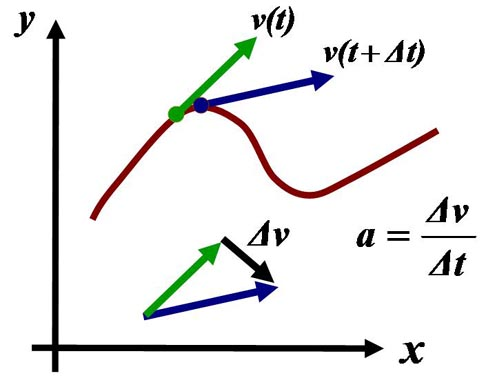Acceleration is the rate of change of velocity. At any point on a trajectory, the magnitude of the acceleration is given by the rate of change of velocity in both magnitude and direction at that point. The true acceleration at time t is found in the limit as time interval Δt → 0.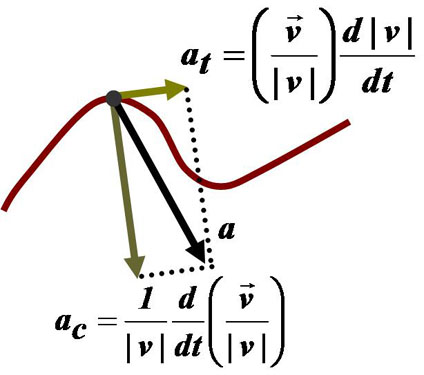Components of acceleration for a planar curved motion. The tangential component at is due to the change in speed of traversal, and points along the curve in the direction of the velocity vector. The centripetal component ac is due to the change in direction of the velocity vector and is normal to the trajectory, pointing toward the center of curvature of the path.

In physics, and more specifically kinematics, acceleration is the change in velocity over time. Because velocity is a vector, it can change in two ways: a change in magnitude and/or a change in direction. In one dimension, acceleration is the rate at which something speeds up or slows down. However, as a vector quantity, acceleration is also the rate at which direction changes. Acceleration has the dimensions L T. In SI units, acceleration is measured in metres per second squared (m/s).

In common speech, the term acceleration commonly is used for an increase in speed (the magnitude of velocity); a decrease in speed is called deceleration. In physics, a change in the direction of velocity also is an acceleration: for motion on a planar surface, the change in direction of velocity results in centripetal acceleration; whereas the rate of change of speed is a tangential acceleration.

In classical mechanics, the acceleration of a body is proportional to the resultant (total) force acting on it (Newton's second law):$\mathbf{F} = m\mathbf{a} \quad \to \quad \mathbf{a} = \mathbf{F}/m$

where F is the resultant force acting on the body, m is the mass of the body, and a is its acceleration.

## Tangential and centripetal acceleration

The velocity of a particle moving on a curved path as a function of time can be written as: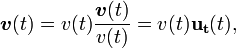$\boldsymbol v (t) =v(t) \frac { \boldsymbol v(t)}{v(t)} = v(t) \mathbf{u_t}(t) ,$

with v(t) equal to the speed of travel along the path, and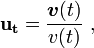$\mathbf{u_t} = \frac {\boldsymbol v( t)}{v(t)} \ ,$

a unit vector tangent to the path pointing in the direction of motion at the chosen moment in time. Taking into account both the changing speed v(t) and the changing direction of ut, the acceleration of a particle moving on a curved path on a planar surface can be written using the chain rule of differentiation as: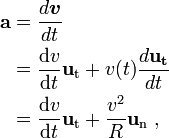\begin{alignat}{3} \mathbf{a} & = \frac{d \boldsymbol v}{dt} \\& =\frac{\mathrm{d}v }{\mathrm{d}t} \mathbf{u}_\mathrm{t} +v(t)\frac{d \mathbf {u_t}}{dt} \\& = \frac{\mathrm{d}v }{\mathrm{d}t} \mathbf{u}_\mathrm{t}+ \frac{v^2}{R}\mathbf{u}_\mathrm{n}\ , \\ \end{alignat}

where un is the unit (outward) normal vector to the particle's trajectory, and R is its instantaneous radius of curvature based upon the osculating circle at time t. These components are called the tangential acceleration and the radial acceleration, respectively. The negative of the radial acceleration is the centripetal acceleration, which points inward, toward the center of curvature.

Extension of this approach to three-dimensional space curves that cannot be contained on a planar surface leads to the Frenet-Serret formulas.

## Relation to relativity

After completing his theory of special relativity, Albert Einstein realized that forces felt by objects undergoing constant proper acceleration are actually feeling themselves being accelerated, so that, for example, a car's acceleration forwards would result in the driver feeling a slight push backwards. In the case of gravity, which Einstein concluded is not actually a force, this is not the case; acceleration due to gravity is not felt by an object in free-fall. This was the basis for his development of general relativity, a relativistic theory of gravity.

This is also the resolution for the proposed twin paradox in special relativity, which asks why one twin ages less when moving away from her sibling at near the speed of light and then returning; the answer being, because the non-aging twin did, in fact, feel acceleration as it was accelerated; this means its velocity changed, and the effects of time dilation on her as well. This cannot be said for the aging, stationary twin, who remained in the same reference frame and aged at a faster pace because of her lesser velocity.

Special relativity applies only to nonaccelerating objects. This is due to the fact that special relativity deals with observers in inertial frames, who must travel at a constant speed in order to measure objects in a manner consistent with special relativity. When an object accelerates, however, it cannot be an observer for any particular reference frame, as its velocity is changing. Gravity, however, is not considered a force, and any object affected only by gravity may be analyzed from a free float reference frame; general relativity applies to it.

## In-line references and notes

Published in July 2009.